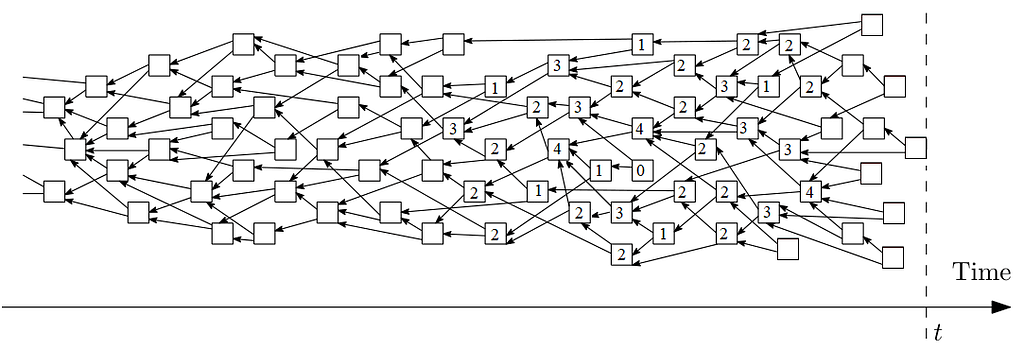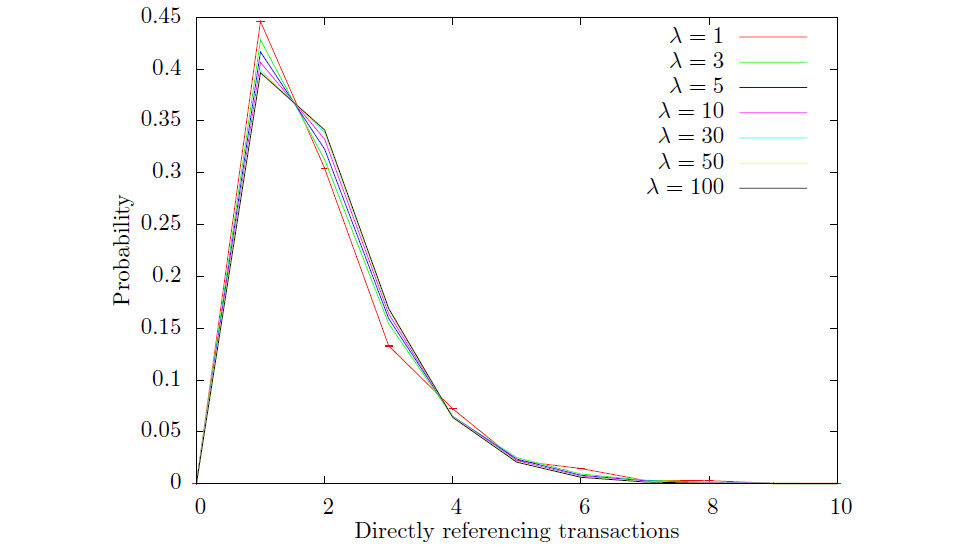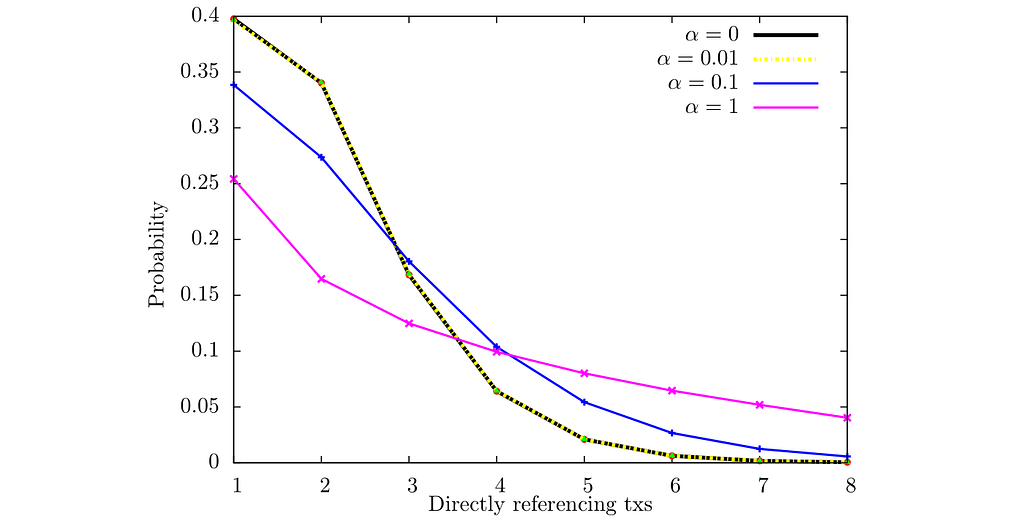# The structure of the Tangle — Number of approvers

In this blog, we talk about the number of direct approvers a tip can receive. In the Tangle, each transaction approves exactly two tips. While this implies that the average number of direct approvers is equal to two, the actual number of approvers can vary significantly between transactions. This is shown in Fig. 1. Here we will look in particular at the probability distribution P(n) to have exactly n approvers. In other words, we are interested in the likely number of potential paths that a random walk will choose from a random transaction. This metric is not only interesting for investigating the structure of the Tangle, but it also allows us to make some probabilistic predictions. For example, recent research showed that the probability distribution P(n) can be employed to make predictions on whether certain parts of the parasite chain attack would be successful, hence providing us with insight under which conditions we should apply caution.Fig. 1 Example of a Tangle. The number inside the square indicates the number of approvers n for a given transaction.

In this blog, we will focus on the tip selection with Markov-Chain-Monte-Carlo random walk with the parameter α set to zero. Although this value does not provide the same security guarantees as the random walk algorithm with larger α does, it is still useful to model the dynamics of the Tangle and can provide useful insights to more generic scenarios. In addition, we will in parts of this analysis also investigate the behavior of the Tangle when using a uniform random tip selection (URTS). This allows us to obtain simpler mathematical expressions that still reproduce some of the dynamics.

Fig. 2 shows P(n) for URTS and α=0 if the tip selection algorithm creates only single links. Hence, if the same tip is selected twice only one connection is created between the approver and the approve. We chose this approach, since it is the simplest, and it should not affect the results in the high load regime if there are no tips left behind. You can see that at low transaction rates it is likely that a transaction has only one approver (see this for more details). On the other hand as λ increases, the probabilities converge towards a constant value. Notice how for URTS the likelihood to have one or two approvers is exactly the same, and that this is not the case for α=0.Fig. 2 P(n) with transaction rate λ for several numbers of approvers n.

We can investigate this distribution in more detail. It is well understood that the distribution should resemble approximately a Poisson distribution, which is demonstrated in Fig. 3. Here we can see what this distribution looks like and how it depends on λ. You can notice that for all λ the likelihood to have a high number of approver drops off very rapidly.Fig. 3 P(n) with the number of approvers n. ( α=0 )

We can also study the behavior of the distribution for α>0, as shown in Fig 4. You can see that for each α>0, as the arrival rate λ increases, the probability of a transaction to have zero approvers becomes non-negligible. However, we can improve this situation through, for example, reattaching transactions or adjusting α appropriately. For the latter, studies like this provide us with information about where we can place the parameter.Fig. 4 P(n) for several values of α.

Once tips are left behind, the distribution P(n) and hence the structure of the Tangle changes. To analyze this scenario, in Fig. 5 we show the probability of having n approvers if tips left behind are ignored, i.e. we don’t consider transactions with zero approvers. As explained in Fig. 4, the value of α could be such that the number of tips left behind is non-negligible. In this case there would be a substantial change in the structure towards a high number of links for an increasingly small number of approved transactions. In effect, a few transactions would be favored over others.Fig. 5 Probability to have n approvers once a transaction receives at least one approver. (λ=50)

As you have seen we can learn a significant amount of information about the Tangle by investigating the aforementioned metrics. However more questions remain open:

• How exactly does the parameter α influence this distribution?
• Should we change the value of α as the transaction rate changes. If so — how?
• How exactly does the number of left-behind tips affect the distribution?
• How do reattachments affect this distribution?

We look forward to keeping you informed about the developments that we find as our research progresses on these fascinating topics. As always we hope you enjoyed the journey through this aspect of our research and we welcome your comments and questions either here or in #tanglemath on our Discord.

The full article was originally published by Andreas Penzkofer on Medium, where people are continuing the conversation by highlighting and responding to this story. Read the full article here.

You might also like

This website uses cookies to improve your experience. We'll assume you're ok with this, but you can opt-out if you wish. AcceptRead More